## Example Questions

### Example Question #3 : Complex Fractions

Add: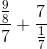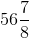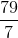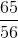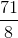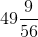Explanation:

To add, first simply each term by rewriting the terms using a division sign.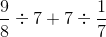Take the reciprocal of the terms after the division sign, and change the division sign to a multiplication sign. Simplify.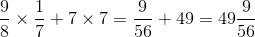### Example Question #4 : Complex Fractions

Add: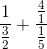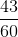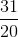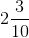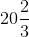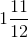Explanation:

The terms shown are complex fractions.  We first need to simplify each and find the least common denominator before solving.

Rewrite the complex fraction using a division sign.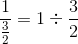Change the division sign to a multiplication sign, and take the reciprocal of the second term.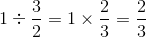Repeat this step for the second term.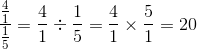The answer is: